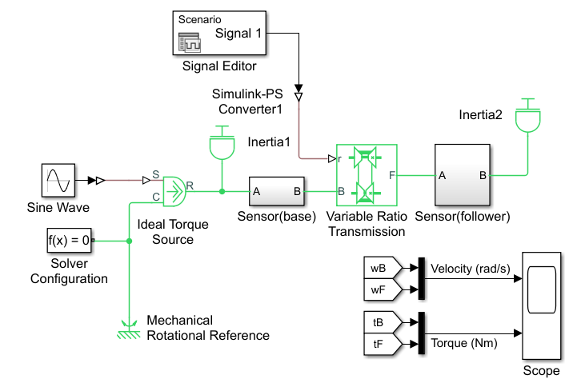## Couple Two Spinning Inertias with a Variable Ratio Transmission

You can modify the gear model from the Couple Two Spinning Inertias with a Simple Gear example by replacing the fixed-ratio gear with a transmission whose gear ratio varies in time. You specify the gear ratio variation with a Simulink® signal converted to a unitless physical signal. Start with the gear model that you built in the Couple Two Spinning Inertias with a Simple Gear example or by opening and editing the `SimpleGearExample` model.

1. From the Simscape™ Driveline™ block library, drag and drop a Variable Ratio Transmission block and replace the Simple Gear block with it. The two shafts spin in the same direction because the Output shaft rotates parameter for the Variable Ratio Transmission block is set to In same direction as input (the default setting).

2. The Variable Ratio Transmission block accepts the continuously varying gear ratio as a physical signal through the extra physical signal input labeled `r`. For this example, create a variable signal for the gear ratio with a Signal Editor block from the Simulink block library and Simulink-PS Converter block. Build a signal that rises with constant slope from 1 to 2 over 10 seconds. Then connect the converted physical signal to the `r` port.

Simple Variable Ratio Transmission Model3. Do not change the other, original settings of the simple gear model. Open the Scope and start the simulation.

The angular velocities and torques of the two shafts have the same signs. The ratios of angular velocities and torques start at 1, because the initial gear ratio is 1. As the gear ratio increases toward 2, the angular velocity of Inertia2 becomes smaller than the velocity of Inertia1, while the associated torque in the second shaft becomes larger than the torque in the first shaft. Because of the changing gear ratio, the motion and the torques are no longer strictly sinusoidal, even though the actuating external torque is.

The Variable Ratio Gear example is a full model of this type. To learn more about how to use variable gear ratios, consult the Variable Ratio Transmission block reference page.